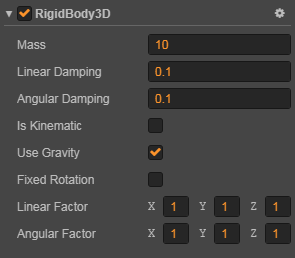# 3D RigidBody Component

RigidBody is the basic object that make up the physical world, and it can make a node physically affected and react.Click the Add Component -> Physics Component -> Rigid Body 3D button at the bottom of the Properties panel to add the RigidBody component to the node.

## RigidBody Properties

Properties Function explanation
Mass The mass of the rigidbody
Linear Damping Used to reduce the linear rate of rigidbody. The larger the value, the slower the rigidbody moves
Angular Damping Used to reduce the rotation rate of rigidbody. The larger the value, the slower the rigidbody rotates
Is Kinematic If enabled, the developer controls the displacement and rotation of the rigidbody, not the physics engine
Use Gravity If enabled, the rigidbody is affected by gravity.
Fixed Rotation If enabled, the rigidbody will be fixed without rotation during a collision.
Linear Factor It can affect the linear velocity change of the rigidbody in each axis. The larger the value, the faster the rigidbody moves.
Angular Factor It can affect the rotation speed change of the rigidbody in each axis. The larger the value, the faster the rigidbody rotates.

For the API interface of RigidBody, please refer to RigidBody3D.

## RigidBody Motion

There are several ways to make a rigidbody move:

• By gravity

Set the `useGravity` property to `true`.

• By force

According to Newton's Second Law `F = m * a`, applying force to a point on the rigidbody will produce acceleration, which will slowly change the velocity of the object over time.

``````rigidBody.applyForce(cc.v3(200, 0, 0));
``````
• By impulse

According to the Momentum Conservation Equation `F * Δt = m * Δv`, when an impulse is applied to a point on the rigidbody, the velocity will change.

``````rigidBody.applyImpulse(cc.v3(5, 0, 0));
``````
• By torque

Force and impulse can also affect the rotation only, causing the rigidbody to rotate. This kind of force is called torque.

``````rigidBody.applyTorque(cc.v3(200, 0, 0));
``````
• By changing the velocity

Using the `setLinearVelocity` to change the line velocity.
Using the `setAngularVelocity` to change the rotation speed.

``````// change the line velocity
rigidBody.setLinearVelocity(cc.v3(5, 0, 0));

// change the rotation speed
rigidBody.setAngularVelocity(cc.v3(5, 0, 0));
``````
• By 3D ConstantForce component

Please refer to the 3D ConstantForce component for details.

## Let rigidbody asleep or awake

### Let rigidbody asleep

If a rigidbody is sleeping, all the force and velocity of the rigidbody are cleared, and the rigidbody freezed.

``````// asleep
if (rigidBody.isAwake) {
rigidBody.sleep();
}
``````

Note: The rigidbody can be woken by force, impulse and change the velocity.

### Let rigidbody awake

``````// awake
if (rigidBody.isSleeping) {
rigidBody.wakeUp();
}
``````

## Restrict RigidBody Motion

There are several ways to restrict a rigidbody move:

• By damping

The `linearDamping` property is used to set the linear damping.
The `angularDamping` property is used to set the angular damping.

The range of the damping parameter is from 0 to infinity, where 0 means no damping and infinity means full damping. Generally, the value of damping is between 0 ~ 0.1.

• By fixed rotation

Set the `fixedRotation` property to `true`.

• By factor

The `linearFactor` property is used to set the linear factor.
The `angularFactor` property is used to set the angular factor.

The factor is type `Vec3`. Value of the corresponding components is used to scale the velocity of corresponding axises. The default values are 1, which means that the scaling are 1x, that is, no scale.

Note: Set a component value of the factor to 0 to fix movement or rotation for a certain axis. If you want to completely fix the rotation, please use `fixedRotation`.